Skip to content
Related Articles
DATEDIF Function in Excel
• Last Updated : 13 Apr, 2021

The DATEDIF function is generally used to find the number of total days, months, and years in between two dates.

### Formula :

There are three formulas to calculate year, month and days:

For the total number of years =datedif(a3,b3,”y”): Where the first argument is the field, and they represent the year.

Example 1: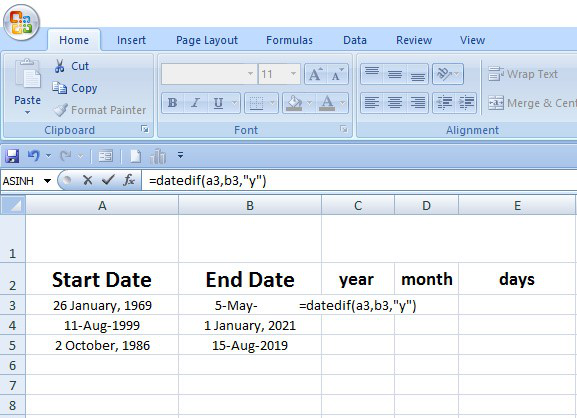Example 2: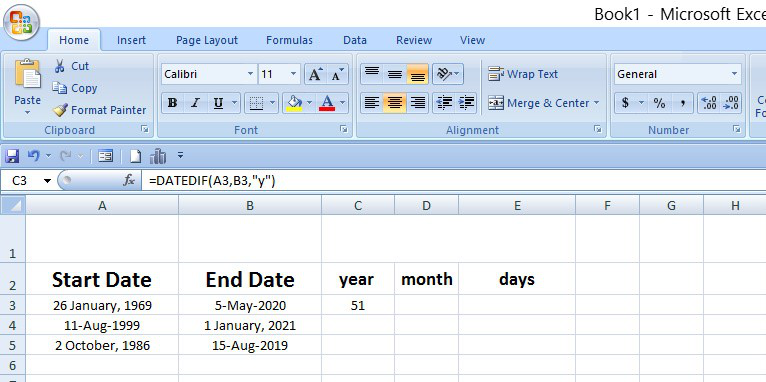For total number of months  =datedif(a3,b3,”m”): Where the first argument is the field and the “m” represents the month.

Example 1: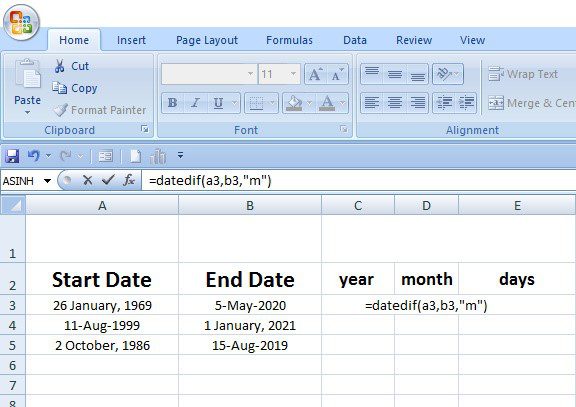Example 2: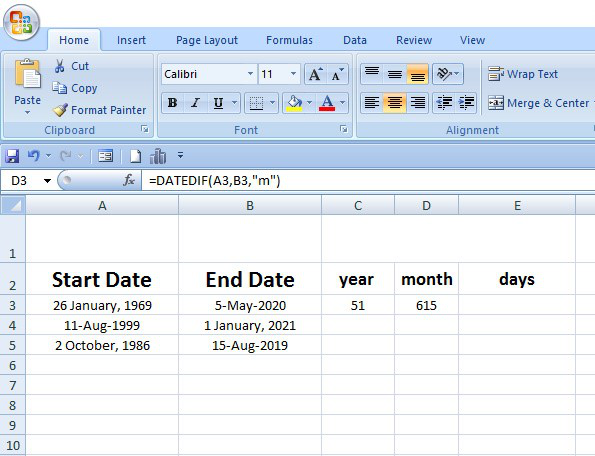For the total number of days   =datedif(a3,b3,”d”): Where the first argument is the field and the d represent the day.

Example 1: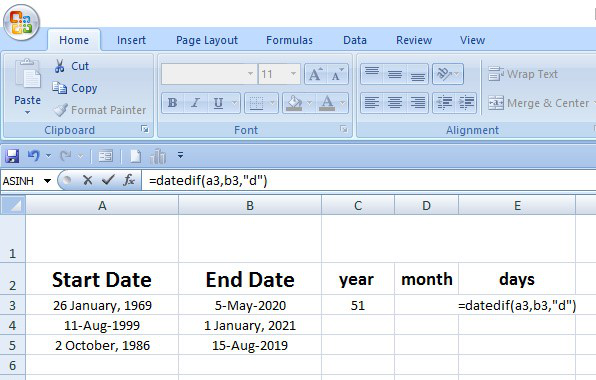Example 2: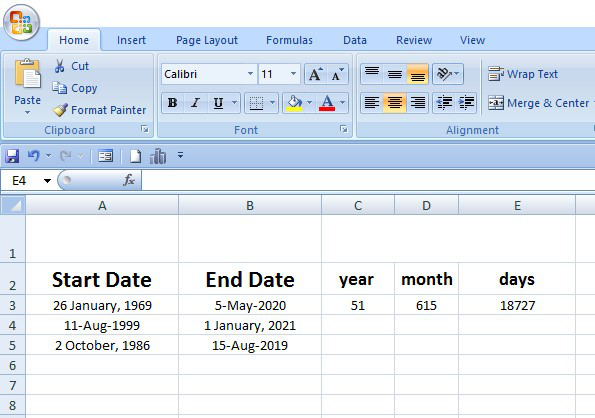### Arguments:

1. The start date is type in DDMMYYYY format. You can select the date format to the home tab from the number.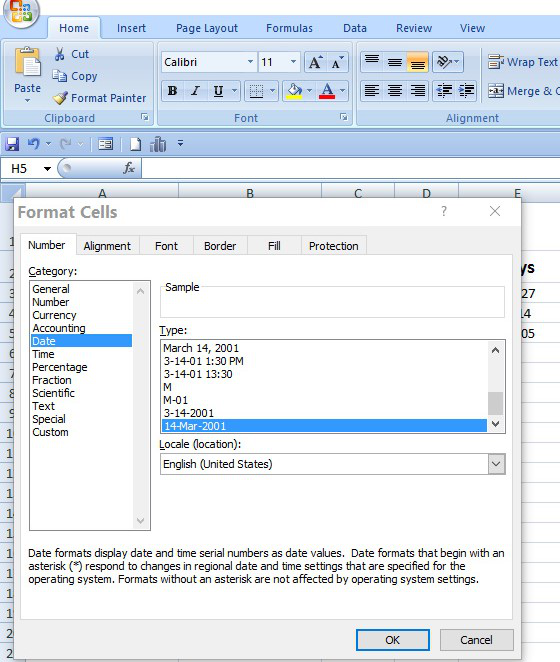2. The last date or end date is type in DDMMYYYY format.

3. The “y” for years unit, “m” for month unit, and “d” for days unit.

4. The sixth type of unit (“y”, “m”,”d”, )  (“ym”,”md”,”yd”)

• “ym” mean number of months without years,  “md” mean number of days  without year and months,
• “yd” means the number of days without years.

Example 1:

By this function, we can find our age (in year months, and days) from our date of birth to the current date. Suppose the date of birth of any person is 26 January 1969 and the current date 05 May 2020 (exact in years months and days ).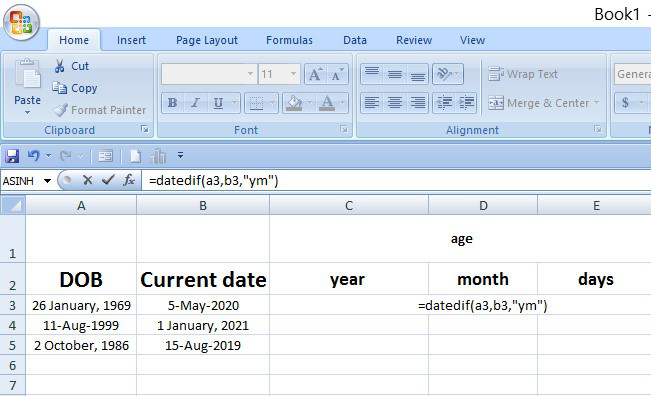Example 2:Example 3: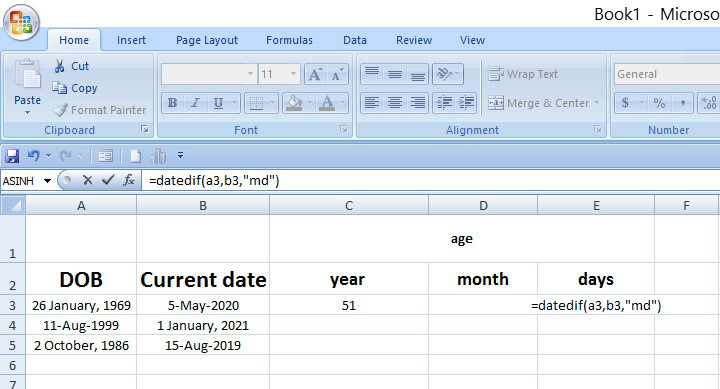Example 4: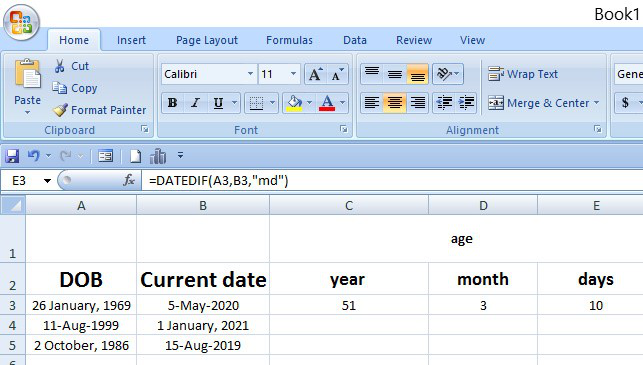My Personal Notes arrow_drop_up